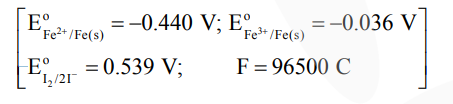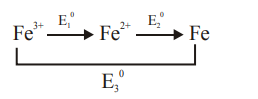Deepak Scored 45->99%ile with Bounce Back Crack Course. You can do it too!

# For the reaction

Question:

For the reaction

$2 \mathrm{Fe}^{3+}(\mathrm{aq})+2 \mathrm{I}^{-}(\mathrm{aq}) \rightarrow 2 \mathrm{Fe}^{2+}(\mathrm{aq})+\mathrm{I}_{2}(\mathrm{~s})$

the magnitude of the standard molar free energy change, $\Delta_{\mathrm{r}} \mathrm{G}_{\mathrm{m}}^{\circ}=-$ $\mathrm{kJ}$ (Round off to the Nearest Integer).Solution:$\mathrm{E}_{1}^{0}+2 \mathrm{E}_{2}^{0}=3 \mathrm{E}_{3}^{0}$

$\mathrm{E}_{1}^{0}=3 \mathrm{E}_{3}^{0}-2 \mathrm{E}_{2}^{0}$

$=3(-0.036)-2(-0.44)$

$=+0.772 \mathrm{~V}$

$\mathrm{E}_{\text {cell }}^{0}=\mathrm{E}_{\mathrm{Fe}^{3+} / \mathrm{Fe}^{2+}}^{0}+\mathrm{E}_{\mathrm{I}^{-} / \mathrm{I}_{2}}^{0}=0.233$

$\Delta_{\mathrm{r}} \mathrm{G}^{0}=-2 \times 96.5 \times 0.233=-45$